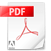tales of future tribes

LA S E R  C U T  A N I M A L I E R  P A T T E R N ,  H A N D  D Y E S  A N D  S L I M  V O L U M E S

C H A R A C T E R I Z E  T H E  " T A L E S  O F  F U T U R E  T R I B E "  C O L L E C T I O N  F O R

T H E  F A L L  W I N T E R  2 1 / 2 2 .

C O R S E T S  AND BELTS M A D E O F T U S C A N Y  V E G E T A B L E

T A N N E D  L E A T H E R ,  T E L L  T H E  W O M A N  O F  T H E  F U T U R E .

R E S I L I E N T  A N D  C O M B A T I V ,  H O N E S T  A N D  F R E E  L I K E  T H E  Q U E E N

O F  A  M E T R O P O L I T A N  T R I B U .TALES OF FUTURE TRIBES
Fall Winter 2021|2022 Collection
LOOKBOOK
tales of future tribes.pdf